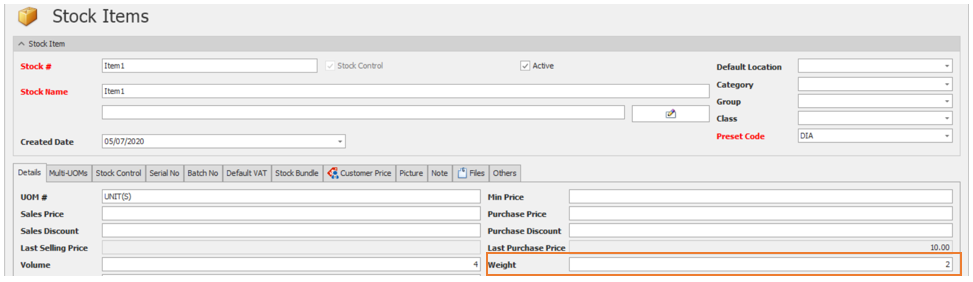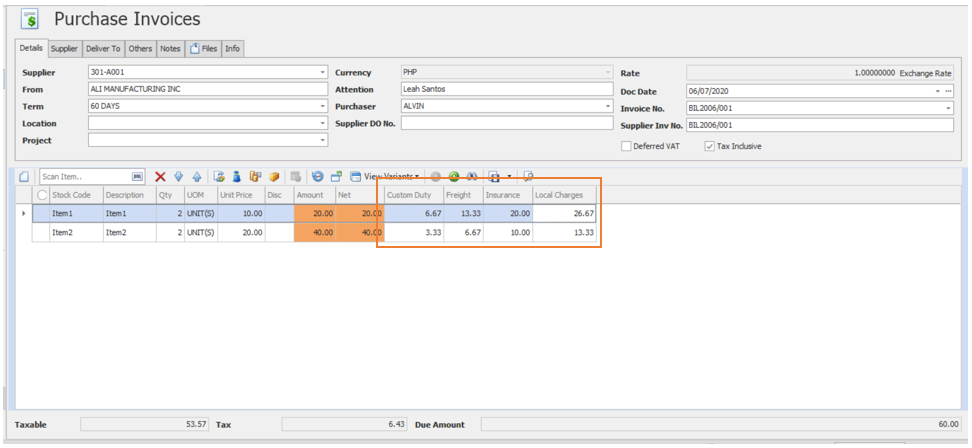# HOW LANDING COST BY WEIGHT WORKS

Modified on Wed, 15 Jun 2022 at 11:38 PM

HOW LANDING COST BY WEIGHT WORKS

Overview:

Another option for computing the Landing Cost is ‘By Weight’. Distribution of cost per Item purchased will be based on the mass/weight. The higher the weight, the higher the amount will be allocated. This method is commonly used for container transportation.

Scenario:

Incorporate the Landed Cost in imported stocks and use weight as reference for allocating the total Landing Cost.

Procedure:

Enable ‘Landing Cost’.

1. Go to Purchases > Landing CostsSet the Proportional into ‘Weight’.Make sure that the Stocks has Weight to use this method.Item1 Weight: 2

Item2 Weight: 1

Application:

Issue a Purchase Invoice and use the Landing cost Calculator as shown below. Input the value of the landing cost.System will automatically compute the Value for Custom Duty, Freight, Insurance, and Local Charges.The calculation of proportion Weight is as below:

 Item1 Custom Duty(Qty x Weight)/Total (Qty x Weight) x Custom Duty = (2x2)/((2x2)+(2x1))x PHP 10 = PHP 6.67 Insurance(Qty x Weight)/Total (Qty x Weight) x Insurance= (2x2)/((2x2) +(2x1)) x PHP 20=  PHP 20 Freight(Qty x Weight)/Total (Qty x Weight) x Freight= (2x2)/((2x2) +(2x1))x PHP 30= PHP 13.33 Local Charges (Qty x Weight)/Total (Qty x Weight) x Local Charges= (2x2)/((2x2) +(2x1)) x PHP 40= PHP 26.67

 Item2 Custom Duty(Qty x Weight)/Total (Qty x Weight) x Custom Duty = (2x1)/((2x2)+(2x1))x 10 php= PHP 3.33 Insurance(Qty x Weight)/Total (Qty x Weight) x Insurance= (2x1)/((2x2) +(2x1)) x PHP 20= 6.67 php Freight(Qty x Weight)/Total (Qty x Weight) x Freight= (2x1)/((2x2) +(2x1))x  PHP 30= PHP 10 Local Charges (Qty x Weight)/Total (Qty x Weight) x Local Charges= (2x1)/((2x2) +(2x1)) x PHP 40= PHP 13.33

 NOTE: